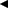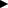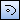# Creating Elliptical Arc

The following 3 methods can be used to create an elliptical arc.

• Specifying the "center point," diameter 1 (vertical radius), diameter 2 (horizontal radius), rotation angle, "start point" and "end point" of an elliptical arc.
• Specifying the "center point," diameter 1 (vertical radius), diameter 2 (horizontal radius), rotation angle, "start angle" and "end angle" of an elliptical arc.
• Specifying the "center point," diameter 1 (vertical radius), diameter 2 (horizontal radius), rotation angle, "start shape" and "end shape" of an elliptical arc.

To display a command parameter to be executed, click/of command parameters, or press Tab key or / key on the keyboard.

1. Click [Elliptical Arc] on [Shape] menu.
Then you will be able to create an elliptical arc.
2.  Supplemental ▪ You can enter into the ellipse creating status by clickingon [Shape] toolbar.
3. Click the center point of an elliptical arc on the drawing.
4. Specify the diameter 1 of an elliptical arc.
When specifying coordinates deciding the length of the diameter 1
Switch the parameter on Command Window to [Point to decide diameter 1] box, and click a point to decide the length of the diameter 1 on the drawing.
The distance between the clicked point and the center point will be the length of the diameter 1.
When specifying the length of the diameter 1
Input the length of the diameter 1 of an elliptical arc in [Diameter 1] box on Command Window.
5. Specify the diameter 2 of an elliptical arc.
When specifying coordinates deciding the length of the diameter 2
Switch the parameter on Command Window to [Point to decide diameter 2] box, and click a point to decide the length of the diameter 2 on the drawing.
The distance between the clicked point and the center point will be the length of the diameter 2.
When specifying the length of the diameter 2
Input the length of the diameter 2 of an elliptical arc in [Diameter 2] box on Command Window.
6.  Supplemental ▪ Among the diameters 1 and 2 specified, the longer one is used as the major radius, while the shorter as the minor radius.
7. Specify the rotation angle for an elliptical arc.
When specifying coordinates for the rotation angle
Switch the parameter on Command Window to [Point to decide rotation angle] box, and click a point to decide the rotation angle.
The clicked point will be specified as the rotation angle for an elliptical arc.
When specifying a rotation angle
Input the rotation angle of an elliptical arc in [Rotation Angle] box on Command Window.
The input angle will be specified as the rotation angle for an elliptical arc.

### When specifying the "start point" and "end point" of an elliptical arc

1. Switch the parameter on Command Window to [Start Point] box, and click the start point of an elliptical arc on the drawing.
2. Switch the parameter on Command Window to [End Point] box, and click the end point of an elliptical arc on the drawing.
An elliptical arc connecting the start and end points will be created.

### When specifying the start and end angles of an elliptical arc

1. Input the start angle for an elliptical arc in [Start Angle] box on Command Window.
2. Input the end angle for an elliptical arc in [End Angle] box on Command Window.
An elliptical arc connecting the start and end angles will be created.

### When selecting the intersection with other shape as the start and end points of an elliptical arc

1. Switch the parameter on Command Window to [Start Shape], and click a shape to be used as the start point.
2. Switch the parameter on Command Window to [End Shape] box, and click a shape to be used as the end point.
An elliptical arc connecting to the intersection with the selected shape will be created.
 Supplemental ▪ You can specify the center point, point to decide diameter 1, point to decide diameter 2, point to decide rotation angle, start point and end point by inputting X and Y coordinates in [Center Point], [Point to decide diameter 1], [Point to decide diameter 2], [Point to decide rotation angle], [Start Point] and [End Point] boxes, respectively.